Office Applications and Entertaiment, Magic SquaresIndex About the Author

6.11   Magic Squares, Misc. Symmetries

6.11.1 Introduction

In previous sections following possible symmetries have been introduced:

• Associated: All pairs of elements, which can be connected with a straight line through the center, sum to 2 * s1 / n
• Complete  : All pairs of elements distant n / 2 along a main - or pan diagonal sum to 2 * s1 / n

and applied in combination with other properties.

For Magic Squares of order 6 these symmetries could only be applied in combination with non consecutive integers (ref. Section 6.09).

Miscellaneous symmetries, which can be applied on 6th order Magic Squares based on consecutive integers (1 .. 36), will be introduced in following sections.

6.11.2 Axial Symmetric Magic Squares

When the equations defining Axial Symmetry as illustrated below:are added to the equations describing a Simple Magic Square of the sixth order (ref. Section 6.2), the resulting Axial Magic Square is described by following set of linear equations:

```a(31) = s1 - a(32) - a(33) - a(34) - a(35) - a(36)
a(25) = s1 - a(26) - a(27) - a(28) - a(29) - a(30)
a(21) =      a(22) - a(26) + a(29) - a(31) + a(36)
a(19) = s1 - a(20) - 2 * a(22) - a(23) - a(24) + a(26) - a(29) + a(31) - a(36)
```
 a(18) = s1/3 - a(24) a(17) = s1/3 - a(23) a(16) = s1/3 - a(22) a(15) = s1/3 - a(21) a(14) = s1/3 - a(20) a(13) = s1/3 - a(19) a(12) = s1/3 - a(30) a(11) = s1/3 - a(29) a(10) = s1/3 - a(28) a( 9) = s1/3 - a(27) a( 8) = s1/3 - a(26) a( 7) = s1/3 - a(25) a(6) = s1/3 - a(36) a(5) = s1/3 - a(35) a(4) = s1/3 - a(34) a(3) = s1/3 - a(33) a(2) = s1/3 - a(32) a(1) = s1/3 - a(31)

The solutions can be obtained by guessing:

a(20), a(22) .. a(24), a(26) .. a(30) and a(32) .. a(36)

and filling out these guesses in the abovementioned equations.

With an optimized guessing routine (MgcSqr6112) numerous Axial Symmetric Magic Squares can be produced.

Attachment 6.11.2 shows the first occuring Axial Symmetric Magic Squares for a(36) = i (i = 1 ... 36).

Note: As discussed in Section 6.10.4 Rectangular Compact Magic Squares might be Axial Symmetric.

6.11.3 Row Symmetric Magic Squares (Type 1)

When the equations defining Row Symmetry as illustrated below: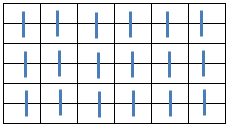are added to the equations describing a Simple Magic Square of the sixth order (ref. Section 6.2), the resulting Row Symmetric Magic Square is described by following set of linear equations:

```a(31) =      s1 - a(32) - a(33) - a(34) - a(35) - a(36)
a(19) =      s1 - a(20) - a(21) - a(22) - a(23) - a(24)
a(11) =           a(12) - a(21) + a(22) - a(31) + a(32)
a( 8) = (2 * s1 - a( 9) - a(10) - 2 * a(12) + 2 * a(21) - 2*a(22) - 2*a(32) - a(33) - a(34) - 2*a(36))/2
a( 7) =           a( 8) - a(21) + a(22) - a(35) + a(36)
```
 a(30) = s1/3 - a(36) a(29) = s1/3 - a(35) a(28) = s1/3 - a(34) a(27) = s1/3 - a(33) a(26) = s1/3 - a(32) a(25) = s1/3 - a(31) a(18) = s1/3 - a(24) a(17) = s1/3 - a(23) a(16) = s1/3 - a(22) a(15) = s1/3 - a(21) a(14) = s1/3 - a(20) a(13) = s1/3 - a(19) a(6) = s1/3 - a(12) a(5) = s1/3 - a(11) a(4) = s1/3 - a(10) a(3) = s1/3 - a( 9) a(2) = s1/3 - a( 8) a(1) = s1/3 - a( 7)

The solutions can be obtained by guessing:

a(9), a(10), a(12), a(20) .. a(24) and a(32) .. a(36)

and filling out these guesses in the abovementioned equations.

With an optimized guessing routine (MgcSqr6113) numerous Row Symmetric Magic Squares can be produced.

Attachment 6.11.3 shows the first occuring Row Symmetric Magic Squares for a(36) = i (i = 1 ... 36).

6.11.4 Row Symmetric Magic Squares (Type 2)

When the equations defining Row Symmetry as illustrated below: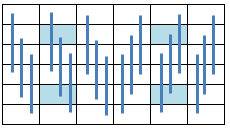are added to the equations describing a Simple Magic Square of the sixth order (ref. Section 6.2), the resulting Row Symmetric Magic Square is described by following set of linear equations:

```a(31) =     s1 - a(32) - a(33) - a(34) - a(35) - a(36)
a(25) =     s1 - a(26) - a(27) - a(28) - a(29) - a(30)
a(21) =   - s1 + a(24) - a(26) + a(29) + a(32) + a(33) + 2*a(34) + a(35) + a(36)
a(20) = 2 * s1 - 2 * a(22) - a(23) - 2*a(24) + 2*a(26) - 2*a(29) - a(32) - 2*a(34) - a(35) - 2*a(36)
a(19) =          a(22) - a(26) + a(29) - a(33) + a(36)
```
 a(18) = s1/3 - a(36) a(17) = s1/3 - a(35) a(16) = s1/3 - a(34) a(15) = s1/3 - a(33) a(14) = s1/3 - a(32) a(13) = s1/3 - a(31) a(12) = s1/3 - a(30) a(11) = s1/3 - a(29) a(10) = s1/3 - a(28) a( 9) = s1/3 - a(27) a( 8) = s1/3 - a(26) a( 7) = s1/3 - a(25) a(6) = s1/3 - a(24) a(5) = s1/3 - a(23) a(4) = s1/3 - a(22) a(3) = s1/3 - a(21) a(2) = s1/3 - a(20) a(1) = s1/3 - a(19)

The solutions can be obtained by guessing:

a(22) .. a(24), a(26) .. a(30) and a(32) .. a(36)

and filling out these guesses in the abovementioned equations.

With an optimized guessing routine (MgcSqr6114) numerous Row Symmetric Magic Squares can be produced.

Attachment 6.11.4 shows the first occuring Row Symmetric Magic Squares for a(36) = i (i = 1 ... 36).

Note: As discussed in Section 6.10.5 Row Symmetric Rectangular Compact Magic Squares don't exist for the Consecutive Integers 1 ... 36.

6.11.5 Crosswise Symmetric Magic Squares
(Non Consecutive Integers)

When the equations defining Crosswise Symmetry as illustrated below:are added to the equations describing a Simple Magic Square of the sixth order (ref. Section 6.2), the resulting Crosswise Symmetric Magic Square can be described by following set of linear equations:

```a(31) =   s1   - a(32) - a(33) - a(34) - a(35) - a(36)
a(19) =   s1   - a(20) - a(21) - a(22) - a(23) - a(24)
a(11) =          a(12) - a(23) + a(24) - a(35) + a(36)
a( 9) =          a(10) - a(21) + a(22) - a(33) + a(34)
a( 8) = 3*s1/2 - a(10) - a(12) - a(20) - a(22) - a(24) - a(32) - a(34) - a(36)
a( 7) =          a( 8) - a(19) + a(20) - a(31) + a(32)
```
 a(30) = s1/3 - a(35) a(29) = s1/3 - a(36) a(28) = s1/3 - a(33) a(27) = s1/3 - a(34) a(26) = s1/3 - a(31) a(25) = s1/3 - a(32) a(18) = s1/3 - a(23) a(17) = s1/3 - a(24) a(16) = s1/3 - a(21) a(15) = s1/3 - a(22) a(14) = s1/3 - a(19) a(13) = s1/3 - a(20) a(6) = s1/3 - a(11) a(5) = s1/3 - a(12) a(4) = s1/3 - a( 9) a(3) = s1/3 - a(10) a(2) = s1/3 - a( 7) a(1) = s1/3 - a( 8)

The solutions require an even Magic Sum s1 and can be obtained by guessing:

a(10), a(12), a(20) .. a(24) and a(32) .. a(36)

and filling out these guesses in the abovementioned equations.

The minimum Magic Sum s1 = 120 occurs for the range {i} = {1 ... 13, 15 ... 19, 21 ... 25, 27 ... 39}.

With an optimized guessing routine (MgcSqr6115) numerous Crosswise Symmetric Magic Squares can be produced.

Attachment 6.11.5 shows the first occuring Crosswise Symmetric Magic Squares for a(36) = i.

6.11.6 Symmetric Magic Squares (Type 3)

When the equations defining the assorted symmetry as illustrated below: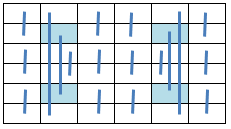are added to the equations describing a Simple Magic Square of the sixth order (ref. Section 6.2), the resulting Symmetric Magic Square can be described by following set of linear equations:

```a(31) =     s1   - a(32) - a(33) - a(34) - a(35) - a(36)
a(26) = 2 * s1/3 - a(29) - a(32) - a(35)
a(19) =     s1   - a(20) - a(21) - a(22) - a(23) - a(24)
a(12) = 2 * s1/3 + a(21) - a(22) - 2 * a(29) + a(31) - a(32) - a(35)
a(9) =      s1   - a(10) - a(31) - a(32) - a(35) - a(36)
a(7) = -2 * s1/3 - a(21) + a(22) + 2 * a(29) + a(32) + a(35) + a(36)
```
 a(30) = s1/3 - a(36) a(28) = s1/3 - a(34) a(27) = s1/3 - a(33) a(25) = s1/3 - a(31) a(18) = s1/3 - a(24) a(17) = s1/3 - a(23) a(16) = s1/3 - a(22) a(15) = s1/3 - a(21) a(14) = s1/3 - a(20) a(13) = s1/3 - a(19) a(11) = s1/3 - a(29) a( 8) = s1/3 - a(26) a(6) = s1/3 - a(12) a(5) = s1/3 - a(35) a(4) = s1/3 - a(10) a(3) = s1/3 - a( 9) a(2) = s1/3 - a(32) a(1) = s1/3 - a( 7)

The solutions can be obtained by guessing:

a(10), a(20) .. a(23), a(29) and a(32) .. a(36)

and filling out these guesses in the abovementioned equations.

With an optimized guessing routine (MgcSqr6116) numerous Symmetric Magic Squares can be produced.

Attachment 6.11.6 shows the first occuring Symmetric Magic Squares for a(36) = i (i = 1 ... 36).

6.11.7 Symmetric Magic Squares (Type 4)

When the equations defining the assorted symmetry as illustrated below: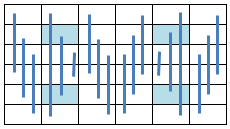are added to the equations describing a Simple Magic Square of the sixth order (ref. Section 6.2), the resulting Symmetric Magic Square can be described by following set of linear equations:

```a(31) = s1 - a(32) - a(33) - a(34) - a(35) - a(36)
a(25) = s1 - a(26) - a(27) - a(28) - a(29) - a(30)
a(22) = s1 - a(24) + a(26) - a(29) - a(32) - a(34) - a(35) - a(36)
a(21) =      a(24) - a(26) + a(29) - a(31) + a(34)
a(20) =    - a(23) + a(32) + a(35)
a(19) =    - a(24) + a(31) + a(36)
```
 a(18) = s1/3 - a(36) a(17) = s1/3 - a(23) a(16) = s1/3 - a(34) a(15) = s1/3 - a(33) a(14) = s1/3 - a(20) a(13) = s1/3 - a(31) a(12) = s1/3 - a(30) a(11) = s1/3 - a(29) a(10) = s1/3 - a(28) a( 9) = s1/3 - a(27) a( 8) = s1/3 - a(26) a( 7) = s1/3 - a(25) a(6) = s1/3 - a(24) a(5) = s1/3 - a(35) a(4) = s1/3 - a(22) a(3) = s1/3 - a(21) a(2) = s1/3 - a(32) a(1) = s1/3 - a(19)

The solutions can be obtained by guessing:

a(23), a(24), a(26) .. a(30) and a(32) .. a(36)

and filling out these guesses in the abovementioned equations.

With an optimized guessing routine (MgcSqr6117) numerous Symmetric Magic Squares can be produced.

Attachment 6.11.7 shows the first occuring Symmetric Magic Squares for a(36) = i (i = 1 ... 36).

6.11.8 Symmetric Magic Squares (Type 5)

When the equations defining the assorted symmetry as illustrated below: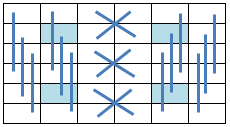are added to the equations describing a Simple Magic Square of the sixth order (ref. Section 6.2), the resulting Symmetric Magic Square can be described by following set of linear equations:

```a(31) =    s1   - a(32) - a(33) - a(34) - a(35) - a(36)
a(25) =  4*s1/3 - a(26) - a(29) - a(30) - a(31) - a(32) - a(35) - a(36)
a(24) =           a(26) - a(29) + a(31)
a(21) =    s1   - a(22) - a(31) - a(32) - a(35) - a(36)
a(20) =         - a(23) + a(32) + a(35)
a(19) =         - a(26) + a(29) + a(36)
a(10) = (2*s1 - 2 * a(22) - 2 * a(31) - 2 * a(32) + a(33) - a(34) - 2*a(35) - 2*a(36))/2
a( 9) =    s1   - a(10) - a(31) - a(32) - a(35) - a(36)
```
 a(28) = s1/3 - a(33) a(27) = s1/3 - a(34) a(18) = s1/3 - a(36) a(17) = s1/3 - a(35) a(16) = s1/3 - a(21) a(15) = s1/3 - a(22) a(14) = s1/3 - a(32) a(13) = s1/3 - a(31) a(12) = s1/3 - a(30) a(11) = s1/3 - a(29) a( 8) = s1/3 - a(26) a( 7) = s1/3 - a(25) a(6) = s1/3 - a(24) a(5) = s1/3 - a(23) a(4) = s1/3 - a( 9) a(3) = s1/3 - a(10) a(2) = s1/3 - a(20) a(1) = s1/3 - a(19)

The solutions can be obtained by guessing:

a(22), a(23), a(26), a(29), a(30), a(32) .. a(36)

and filling out these guesses in the abovementioned equations.

With an optimized guessing routine (MgcSqr6118) numerous Symmetric Magic Squares can be produced.

Attachment 6.11.8 shows the first occuring Symmetric Magic Squares for a(36) = i (i = 1 ... 36).

6.11.9 Symmetric Magic Squares (Type 6)

When the equations defining the assorted symmetry as illustrated below: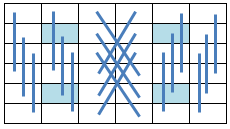are added to the equations describing a Simple Magic Square of the sixth order (ref. Section 6.2), the resulting Symmetric Magic Square can be described by following set of linear equations:

```a(31) =  s1 - a(32) - a(33) - a(34) - a(35) - a(36)
a(25) =  s1 - a(26) - a(27) - a(28) - a(29) - a(30)
a(22) = -s1 + a(24) - a(26) + a(27) - a(28) + a(29) + a(32) + 3 * a(33) + a(35) + a(36)
a(21) = -s1 + a(24) - a(26) + a(29) + a(32) + 2 * a(33) + a(34) + a(35) + a(36)
a(20) = - 4 * a(21) - a(23) - 2 * a(27) + 2 * a(28) + a(32) + 4 * a(34) + a(35)
a(19) = -s1 + a(24) - 2 * a(26) + a(27) - a(28) + 2 * a(29) + a(32) + 3 * a(33) - a(34) + a(35) + 2*a(36)
```
 a(18) = s1/3 - a(36) a(17) = s1/3 - a(35) a(16) = s1/3 - a(33) a(15) = s1/3 - a(34) a(14) = s1/3 - a(32) a(13) = s1/3 - a(31) a(12) = s1/3 - a(30) a(11) = s1/3 - a(29) a(10) = s1/3 - a(27) a( 9) = s1/3 - a(28) a( 8) = s1/3 - a(26) a( 7) = s1/3 - a(25) a(6) = s1/3 - a(24) a(5) = s1/3 - a(23) a(4) = s1/3 - a(21) a(3) = s1/3 - a(22) a(2) = s1/3 - a(20) a(1) = s1/3 - a(19)

The solutions can be obtained by guessing:

a(22) .. a(24), a(26) .. a(30), a(32) .. a(36)

and filling out these guesses in the abovementioned equations.

With an optimized guessing routine (MgcSqr6119) numerous Symmetric Magic Squares can be produced.

Attachment 6.11.9 shows the first occuring Symmetric Magic Squares for a(36) = i (i = 1 ... 36).

6.11.10 Summary

The obtained results regarding the miscellaneous types of order 6 Magic Squares as deducted and discussed in previous sections are summarized in following table:

 Type Characteristics Subroutine Results Simple Axial Symmetric Row Symmetric, Type 1 Row Symmetric, Type 2 Crosswise Symmetric Mixed Symmetric, Type 3 Mixed Symmetric, Type 4 Mixed Symmetric, Type 5 Mixed Symmetric, Type 6
 Comparable routines as listed above, can be used to generate order 6 Pan Magic Squares for non consecutive integers, which will be described in following sections.